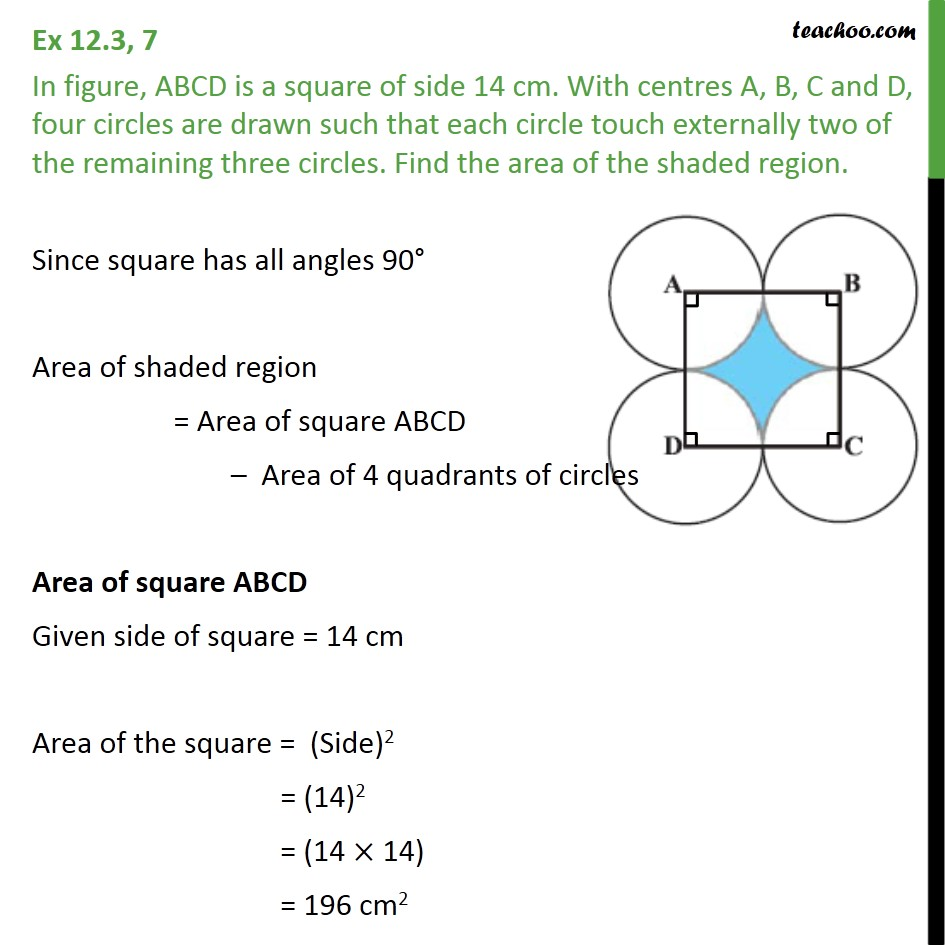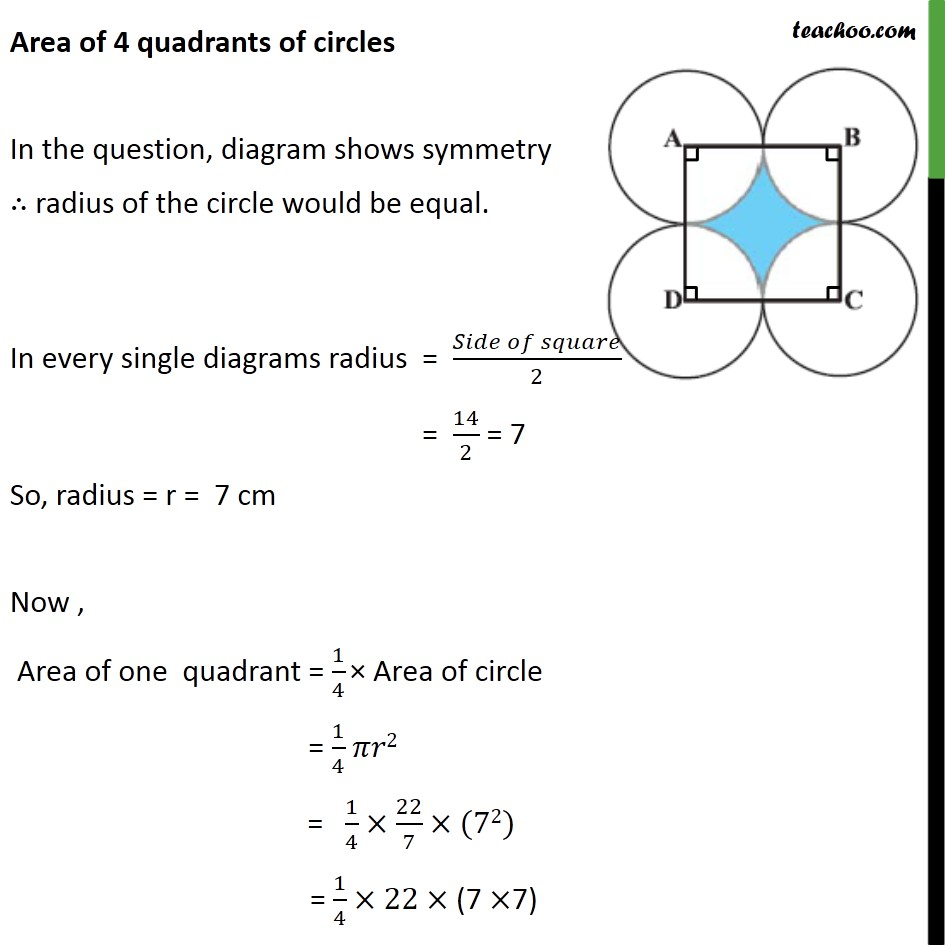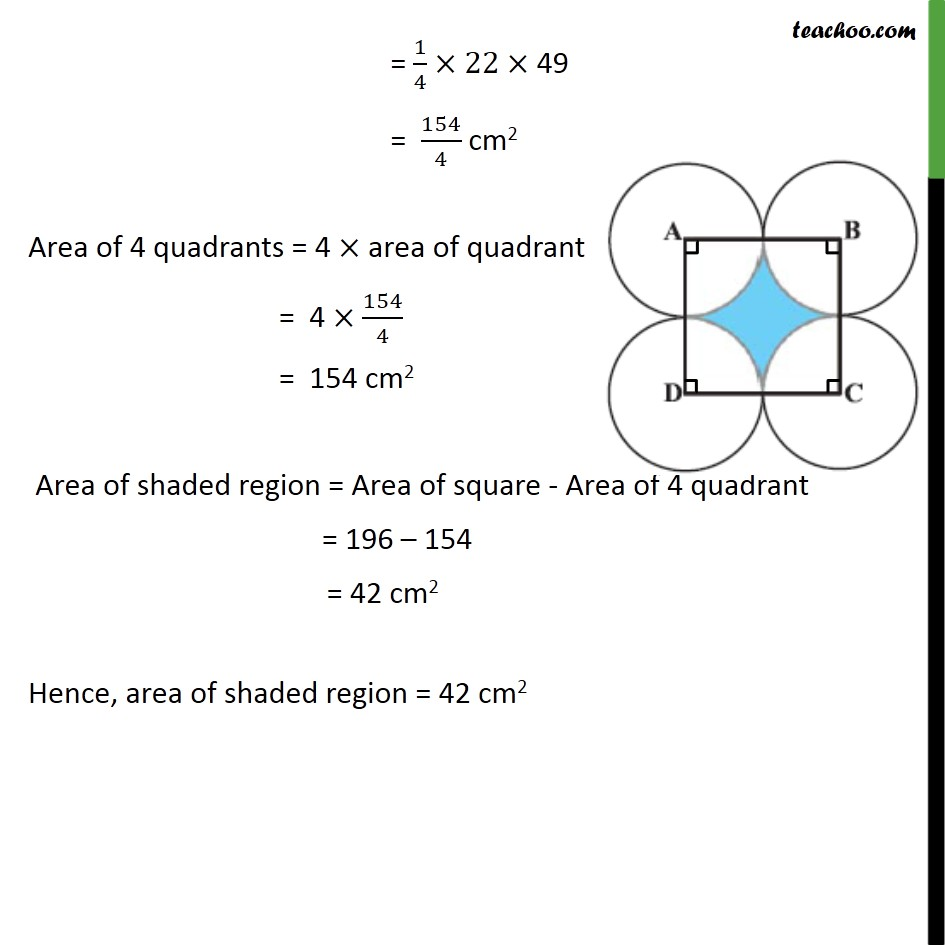1. Chapter 12 Class 10 Areas related to Circles
2. Serial order wise
3. Ex 12.3

Transcript

Ex 12.3, 7 In figure, ABCD is a square of side 14 cm. With centres A, B, C and D, four circles are drawn such that each circle touch externally two of the remaining three circles. Find the area of the shaded region. Since square has all angles 90 Area of shaded region = Area of square ABCD Area of 4 quadrants of circles Area of square ABCD Given side of square = 14 cm Area of the square = (Side)2 = (14)2 = (14 14) = 196 cm2 Area of 4 quadrants of circles In the question, diagram shows symmetry radius of the circle would be equal. In every single diagrams radius = ( )/2 = 14/2 = 7 So, radius = r = 7 cm Now , Area of one quadrant = 1/4 Area of circle = 1/4 2 = 1/4 22/7 (72) = 1/4 22 (7 7) = 1/4 22 49 = 154/4 cm2 Area of 4 quadrants = 4 area of quadrant = 4 154/4 = 154 cm2 Area of shaded region = Area of square - Area of 4 quadrant = 196 154 = 42 cm2 Hence, area of shaded region = 42 cm2

Ex 12.3

About the AuthorDavneet Singh
Davneet Singh is a graduate from Indian Institute of Technology, Kanpur. He has been teaching from the past 9 years. He provides courses for Maths and Science at Teachoo.